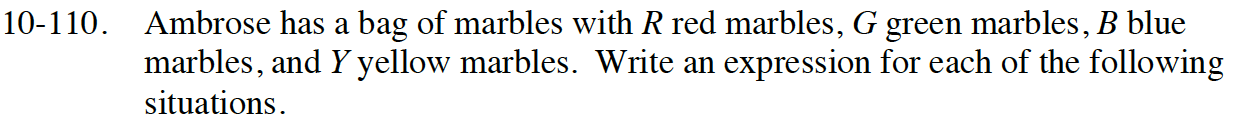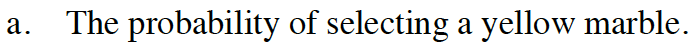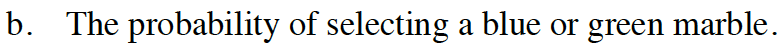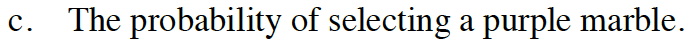### Home > CC4 > Chapter 10 > Lesson 10.2.3 > Problem10-110

10-110.
1. Ambrose has a bag of marbles with R red marbles, G green marbles, B blue marbles, and Y yellow marbles. Write an expression for each of the following situations. Homework Help ✎

1. The probability of selecting a yellow marble.

2. The probability of selecting a blue or green marble.

3. The probability of selecting a purple marble.$\frac{Y}{R+G+B+Y}$Add the blue and green together.How many purple marbles are there?